Math resources Geometry Lines

Parallel lines

# Parallel lines

Here you will learn about parallel lines, including what they are and how to identify them.

Students will first learn about parallel lines as part of geometry in 4 th grade. They continue to build on this knowledge in 7 th grade geometry.

## What are parallel lines?

Parallel lines are lines that never intersect because they are always the same distance apart.

For example,

Parallel lines are seen in many common 2D shapes.

For example,

Each side of a square is made of a line segment that is part of a line. The opposite sides of a square are parallel.

There are also many examples of parallel lines in real life.

For example,

The lines that pass by the sides of a table top are parallel.

When a line passes through a set of parallel lines, it is a transversal line.

For example,

Parallel lines cut by a transversal, create 8 angles. The corresponding angles, vertical angles, alternate exterior angles and alternate interior angles created are examples of congruent pairs of angles. The consecutive interior angles, consecutive exterior angles and the adjacent angles of the side of the transversal are examples of supplementary pairs of angles.

### What are parallel lines?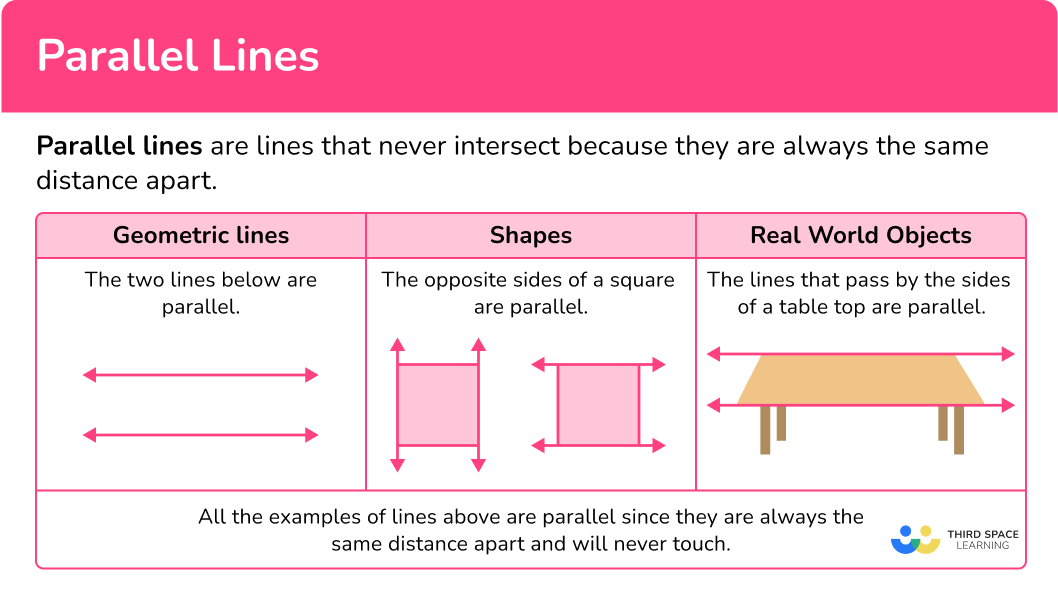## Common Core State Standards

How does this relate to 4 th grade math?

• 4th Grade – Geometry (4.G.A.1)
Draw points, lines, line segments, rays, angles (right, acute, obtuse), and perpendicular and parallel lines. Identify these in two-dimensional figures.

## How to identify parallel lines

In order to identify parallel lines:

1. Look for two lines that do not intersect.
2. Decide if the lines are always the same distance apart.

## Parallel lines examples

### Example 1: two lines crossing

1. Look for two lines that do not intersect.

The image shows two intersecting lines (lines that cross), which does not meet the parallel lines definition.

The lines are not parallel.

### Example 2: geometric lines

Do line AB and line CD appear to be parallel?

The image shows two lines, line AB and line CD , that do not intersect (cross).

The lines look as if they are always the same distance apart, so line AB and line CD appear to be parallel.

### Example 3: parallel lines in a rectangle

Highlight any sets of parallel lines in the rectangle.

Each side of a rectangle is made of a line segment that is part of a line. The opposite sides of the rectangle have lines that do not intersect.

Since a rectangle has four right angles (90^{\circ}) , the lines that make up the corners are perpendicular. This means that the opposite lines will always be the same distance apart. So both sets of lines shown are parallel lines.

### Example 4: lines in a triangle

Highlight any sets of parallel lines in the triangle.

Each side of a triangle is made of a line segment that is part of a line. These lines cross at the vertices of the triangle.

Since all of the lines that make up the triangle intersect, there are NO parallel lines in the triangle.

### Example 5: parallel lines in the real world

Find a set of parallel lines in the door.

There are many lines that do not intersect. An example is the lines that pass by the sides of the door:

The distance does not appear to be changing between them and doors typically have sides that are straight up and down, so we can conclude that they are likely parallel.

The picture below shows all the other sets of parallel lines:

It appears that all the blue lines are parallel to each other and all the red lines are parallel to each other.

### Example 6: parallel lines in the real world

Find a set of parallel lines in the railway tracks.

The railway tracks can be represented by straight lines. There are many lines that do not intersect. An example is:

The distance does not appear to be changing between them and the train wheels traveling on the tracks are always the same distance apart, so we can conclude that they are likely parallel.

The distance does not appear to be changing between them and the train wheels traveling on the tracks are always the same distance apart, so we can conclude that they are likely parallel.

The picture below shows all the other sets of parallel lines:

It appears that all the blue lines are parallel to each other and all the red lines are parallel to each other.

### Teaching tips for parallel lines

• Worksheets that have a mixture of 2D shapes and real world pictures can be useful for this skill, along with everyday 2D and 3D objects that are in the classroom. Encourage students to look for and identify parallel lines everywhere they go. Something as simple as noticing the railings on the steps at school, can be a teaching moment for parallel lines.

• Go beyond just asking students to identify parallel lines, by asking them to create and explain parallel lines in a variety of scenarios. This allows students to test and explain their knowledge of the topic on multiple levels.

### Easy mistakes to make

• Confusing parallel and perpendicular lines
Since they both involve lines, it is easy to get them confused. With enough practice and real world application, students will learn the difference. You can also teach students to remember that the two ls in parallel, will never touch and are therefore parallel to each other.

• Getting confused when more than two lines are shown
It can be hard to figure out the relationship of just two lines at a time when many are shown. Encourage students to highlight the two lines or to use a straight object (like a ruler) to better visualize how they extend.
For example,

Students can…
Highlight two lines:

Extend the lines with an object:

### Practice parallel lines questions

1) Which of the given lines look parallel?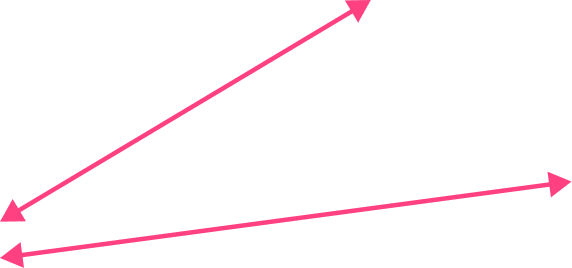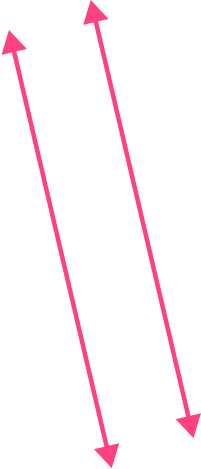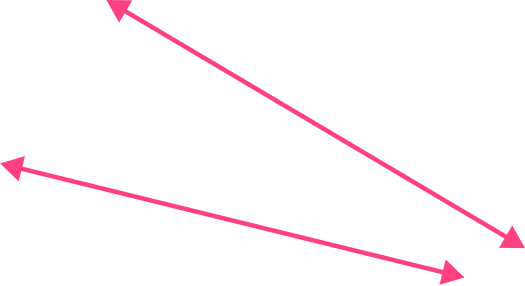The image shows two lines that do not intersect (cross). The lines look as if they are always the same distance apart, so they look parallel.

2) Which of the given lines look parallel to each other?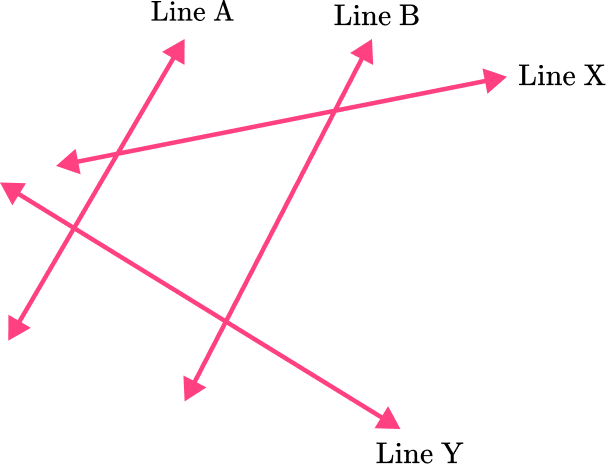line A and line Bline B and line Xline A and line Xline X and line Y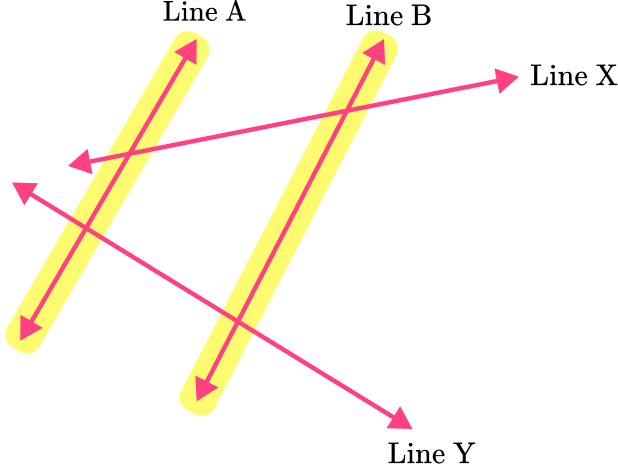Line A and line B do not cross and appear to always be the same distance apart, so they look parallel.

3) Which shape has a pair of parallel lines?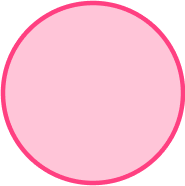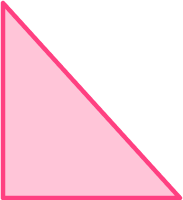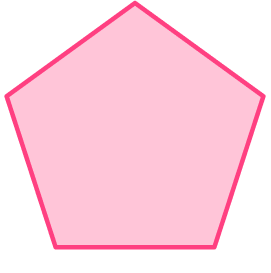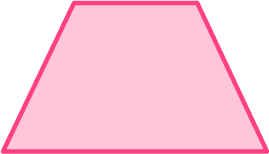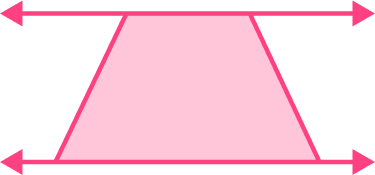The top and bottom of the trapezoid are created by line segments that are part of lines. The two lines do not intersect and are always the same distance apart – making them parallel.

4) Which shape DOES NOT have parallel lines?The lines that make up this triangle all intersect, so they are not parallel.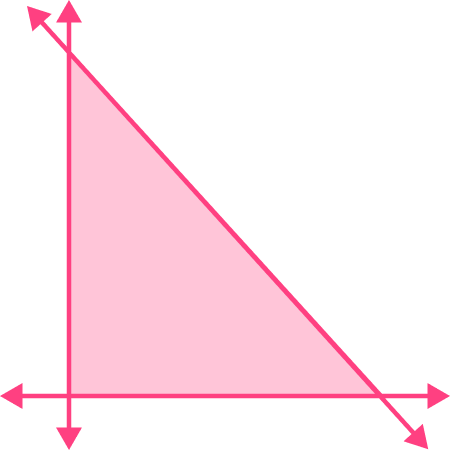This triangle DOES NOT have parallel lines.

5) Which lines on the ice cream look parallel?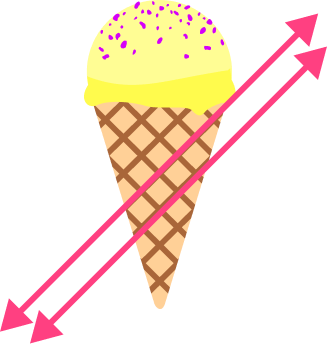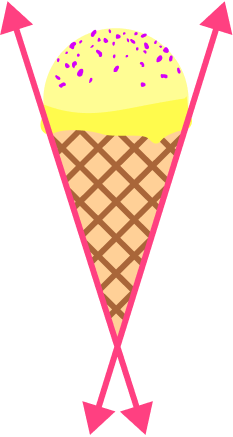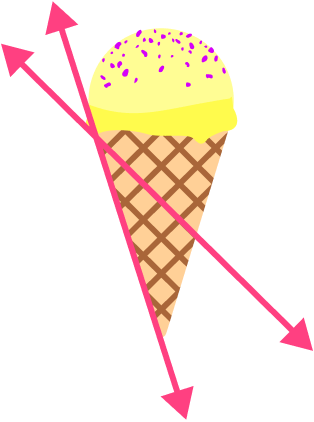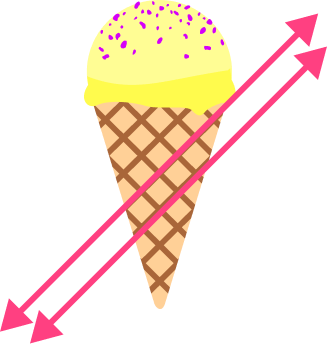The lines do not cross and appear to always be the same distance apart, so they look parallel.

6) Which lines on the barn look parallel?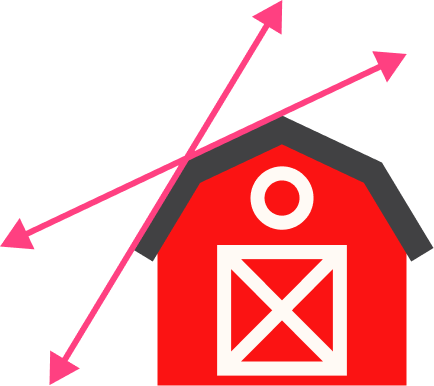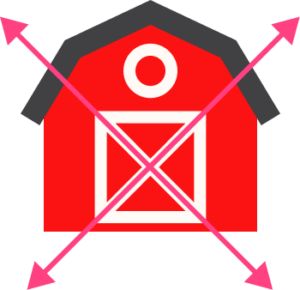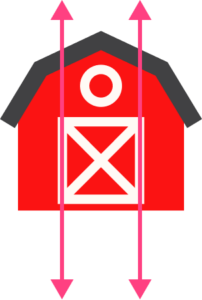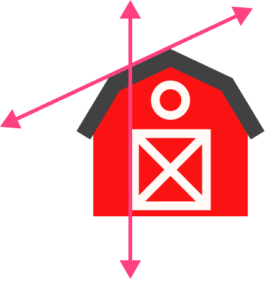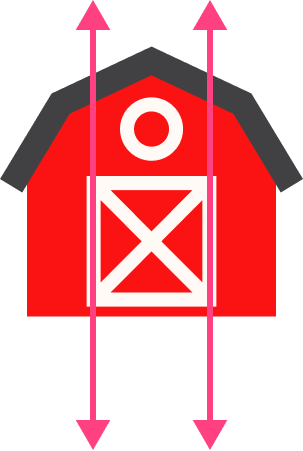The lines do not cross and appear to always be the same distance apart, so they look parallel.

## Parallel lines FAQs

What are the properties of parallel lines?

Parallel lines are always straight lines that are the same distance apart, they never intersect and they are coplanar (meaning on the same plane).

How are parallel lines used on a coordinate plane?

When parallel lines are used on a coordinate plane, an equation of a line can be written for each of them ( y=m x+b ). The linear equations of the parallel lines will include a y-intercept and the slope of a line. Since the lines will never cross, the slopes of parallel lines are the same. This means the parallel lines equations will have the same value for m .

Is a pair of corresponding angles two angles that are supplementary or congruent?

A pair of corresponding angles are equal to each other, so they are not supplementary unless they are both 90 -degree angles.

Is a pair of alternate interior angles two angles that are supplementary or congruent?

A pair of alternate interior angles are equal to each other, so they are not supplementary unless they are both 90 -degree angles.

## Still stuck?

At Third Space Learning, we specialize in helping teachers and school leaders to provide personalized math support for more of their students through high-quality, online one-on-one math tutoring delivered by subject experts.

Each week, our tutors support thousands of students who are at risk of not meeting their grade-level expectations, and help accelerate their progress and boost their confidence.